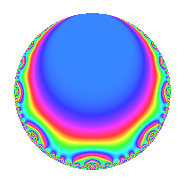# Properties

 Label 8040.2.a.mLevel 8040 Weight 2 Character orbit 8040.a Self dual Yes Analytic conductor 64.200 Analytic rank 0 Dimension 2 CM No Inner twists 1

# Related objects

## Newspace parameters

 Level: $$N$$ = $$8040 = 2^{3} \cdot 3 \cdot 5 \cdot 67$$ Weight: $$k$$ = $$2$$ Character orbit: $$[\chi]$$ = 8040.a (trivial)

## Newform invariants

 Self dual: Yes Analytic conductor: $$64.1997232251$$ Analytic rank: $$0$$ Dimension: $$2$$ Coefficient field: $$\Q(\sqrt{17})$$ Coefficient ring: $$\Z[a_1, \ldots, a_{7}]$$ Coefficient ring index: $$1$$ Fricke sign: $$-1$$ Sato-Tate group: $\mathrm{SU}(2)$

## $q$-expansion

Coefficients of the $$q$$-expansion are expressed in terms of $$\beta = \frac{1}{2}(1 + \sqrt{17})$$. We also show the integral $$q$$-expansion of the trace form.

 $$f(q)$$ $$=$$ $$q$$ $$+ q^{3}$$ $$+ q^{5}$$ $$+ \beta q^{7}$$ $$+ q^{9}$$ $$+O(q^{10})$$ $$q$$ $$+ q^{3}$$ $$+ q^{5}$$ $$+ \beta q^{7}$$ $$+ q^{9}$$ $$+ ( 4 - \beta ) q^{11}$$ $$+ 2 \beta q^{13}$$ $$+ q^{15}$$ $$+ ( -4 + 2 \beta ) q^{17}$$ $$+ ( 4 - 2 \beta ) q^{19}$$ $$+ \beta q^{21}$$ $$-4 q^{23}$$ $$+ q^{25}$$ $$+ q^{27}$$ $$+ ( 2 + 2 \beta ) q^{29}$$ $$+ ( 2 + 2 \beta ) q^{31}$$ $$+ ( 4 - \beta ) q^{33}$$ $$+ \beta q^{35}$$ $$+ ( 2 - 3 \beta ) q^{37}$$ $$+ 2 \beta q^{39}$$ $$+ 6 q^{41}$$ $$+ ( 8 - 2 \beta ) q^{43}$$ $$+ q^{45}$$ $$-2 \beta q^{47}$$ $$+ ( -3 + \beta ) q^{49}$$ $$+ ( -4 + 2 \beta ) q^{51}$$ $$+ ( -2 + 2 \beta ) q^{53}$$ $$+ ( 4 - \beta ) q^{55}$$ $$+ ( 4 - 2 \beta ) q^{57}$$ $$+ ( -2 - 4 \beta ) q^{59}$$ $$+ ( -2 + 3 \beta ) q^{61}$$ $$+ \beta q^{63}$$ $$+ 2 \beta q^{65}$$ $$+ q^{67}$$ $$-4 q^{69}$$ $$+ ( 10 - \beta ) q^{71}$$ $$+ ( -6 - 2 \beta ) q^{73}$$ $$+ q^{75}$$ $$+ ( -4 + 3 \beta ) q^{77}$$ $$-2 q^{79}$$ $$+ q^{81}$$ $$+ ( -12 + \beta ) q^{83}$$ $$+ ( -4 + 2 \beta ) q^{85}$$ $$+ ( 2 + 2 \beta ) q^{87}$$ $$+ ( 10 - 5 \beta ) q^{89}$$ $$+ ( 8 + 2 \beta ) q^{91}$$ $$+ ( 2 + 2 \beta ) q^{93}$$ $$+ ( 4 - 2 \beta ) q^{95}$$ $$+ ( 12 + \beta ) q^{97}$$ $$+ ( 4 - \beta ) q^{99}$$ $$+O(q^{100})$$ $$\operatorname{Tr}(f)(q)$$ $$=$$ $$2q$$ $$\mathstrut +\mathstrut 2q^{3}$$ $$\mathstrut +\mathstrut 2q^{5}$$ $$\mathstrut +\mathstrut q^{7}$$ $$\mathstrut +\mathstrut 2q^{9}$$ $$\mathstrut +\mathstrut O(q^{10})$$ $$2q$$ $$\mathstrut +\mathstrut 2q^{3}$$ $$\mathstrut +\mathstrut 2q^{5}$$ $$\mathstrut +\mathstrut q^{7}$$ $$\mathstrut +\mathstrut 2q^{9}$$ $$\mathstrut +\mathstrut 7q^{11}$$ $$\mathstrut +\mathstrut 2q^{13}$$ $$\mathstrut +\mathstrut 2q^{15}$$ $$\mathstrut -\mathstrut 6q^{17}$$ $$\mathstrut +\mathstrut 6q^{19}$$ $$\mathstrut +\mathstrut q^{21}$$ $$\mathstrut -\mathstrut 8q^{23}$$ $$\mathstrut +\mathstrut 2q^{25}$$ $$\mathstrut +\mathstrut 2q^{27}$$ $$\mathstrut +\mathstrut 6q^{29}$$ $$\mathstrut +\mathstrut 6q^{31}$$ $$\mathstrut +\mathstrut 7q^{33}$$ $$\mathstrut +\mathstrut q^{35}$$ $$\mathstrut +\mathstrut q^{37}$$ $$\mathstrut +\mathstrut 2q^{39}$$ $$\mathstrut +\mathstrut 12q^{41}$$ $$\mathstrut +\mathstrut 14q^{43}$$ $$\mathstrut +\mathstrut 2q^{45}$$ $$\mathstrut -\mathstrut 2q^{47}$$ $$\mathstrut -\mathstrut 5q^{49}$$ $$\mathstrut -\mathstrut 6q^{51}$$ $$\mathstrut -\mathstrut 2q^{53}$$ $$\mathstrut +\mathstrut 7q^{55}$$ $$\mathstrut +\mathstrut 6q^{57}$$ $$\mathstrut -\mathstrut 8q^{59}$$ $$\mathstrut -\mathstrut q^{61}$$ $$\mathstrut +\mathstrut q^{63}$$ $$\mathstrut +\mathstrut 2q^{65}$$ $$\mathstrut +\mathstrut 2q^{67}$$ $$\mathstrut -\mathstrut 8q^{69}$$ $$\mathstrut +\mathstrut 19q^{71}$$ $$\mathstrut -\mathstrut 14q^{73}$$ $$\mathstrut +\mathstrut 2q^{75}$$ $$\mathstrut -\mathstrut 5q^{77}$$ $$\mathstrut -\mathstrut 4q^{79}$$ $$\mathstrut +\mathstrut 2q^{81}$$ $$\mathstrut -\mathstrut 23q^{83}$$ $$\mathstrut -\mathstrut 6q^{85}$$ $$\mathstrut +\mathstrut 6q^{87}$$ $$\mathstrut +\mathstrut 15q^{89}$$ $$\mathstrut +\mathstrut 18q^{91}$$ $$\mathstrut +\mathstrut 6q^{93}$$ $$\mathstrut +\mathstrut 6q^{95}$$ $$\mathstrut +\mathstrut 25q^{97}$$ $$\mathstrut +\mathstrut 7q^{99}$$ $$\mathstrut +\mathstrut O(q^{100})$$

## Embeddings

For each embedding $$\iota_m$$ of the coefficient field, the values $$\iota_m(a_n)$$ are shown below.

For more information on an embedded modular form you can click on its label.

Label $$\iota_m(\nu)$$ $$a_{2}$$ $$a_{3}$$ $$a_{4}$$ $$a_{5}$$ $$a_{6}$$ $$a_{7}$$ $$a_{8}$$ $$a_{9}$$ $$a_{10}$$
1.1
 −1.56155 2.56155
0 1.00000 0 1.00000 0 −1.56155 0 1.00000 0
1.2 0 1.00000 0 1.00000 0 2.56155 0 1.00000 0
 $$n$$: e.g. 2-40 or 990-1000 Significant digits: Format: Complex embeddings Normalized embeddings Satake parameters Satake angles

## Inner twists

This newform does not admit any (nontrivial) inner twists.

## Atkin-Lehner signs

$$p$$ Sign
$$2$$ $$1$$
$$3$$ $$-1$$
$$5$$ $$-1$$
$$67$$ $$-1$$

## Hecke kernels

This newform can be constructed as the intersection of the kernels of the following linear operators acting on $$S_{2}^{\mathrm{new}}(\Gamma_0(8040))$$:

 $$T_{7}^{2}$$ $$\mathstrut -\mathstrut T_{7}$$ $$\mathstrut -\mathstrut 4$$ $$T_{11}^{2}$$ $$\mathstrut -\mathstrut 7 T_{11}$$ $$\mathstrut +\mathstrut 8$$ $$T_{13}^{2}$$ $$\mathstrut -\mathstrut 2 T_{13}$$ $$\mathstrut -\mathstrut 16$$ $$T_{17}^{2}$$ $$\mathstrut +\mathstrut 6 T_{17}$$ $$\mathstrut -\mathstrut 8$$